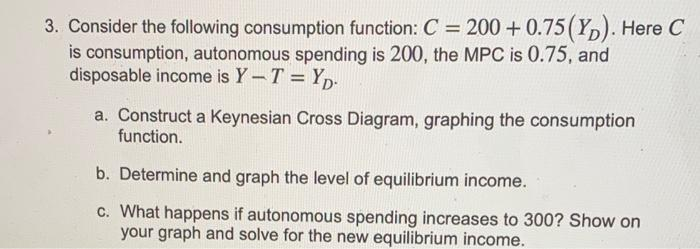# Question Solved1 Answer3. Consider the following consumption function: $$C=200+0.75\left(Y_{D}\right)$$. Here $$C$$ is consumption, autonomous spending is 200 , the MPC is $$0.75$$, and disposable income is $$Y-T=Y_{D}$$. a. Construct a Keynesian Cross Diagram, graphing the consumption function. b. Determine and graph the level of equilibrium income. c. What happens if autonomous spending increases to 300 ? Show on your graph and solve for the new equilibrium income.Transcribed Image Text: 3. Consider the following consumption function: $$C=200+0.75\left(Y_{D}\right)$$. Here $$C$$ is consumption, autonomous spending is 200 , the MPC is $$0.75$$, and disposable income is $$Y-T=Y_{D}$$. a. Construct a Keynesian Cross Diagram, graphing the consumption function. b. Determine and graph the level of equilibrium income. c. What happens if autonomous spending increases to 300 ? Show on your graph and solve for the new equilibrium income.
More
Transcribed Image Text: 3. Consider the following consumption function: $$C=200+0.75\left(Y_{D}\right)$$. Here $$C$$ is consumption, autonomous spending is 200 , the MPC is $$0.75$$, and disposable income is $$Y-T=Y_{D}$$. a. Construct a Keynesian Cross Diagram, graphing the consumption function. b. Determine and graph the level of equilibrium income. c. What happens if autonomous spending increases to 300 ? Show on your graph and solve for the new equilibrium income.